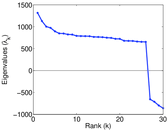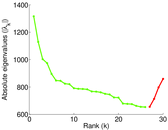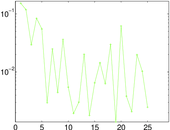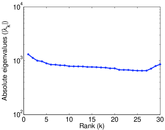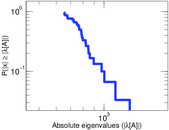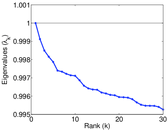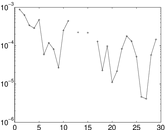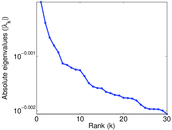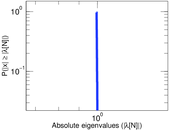# DBLP

This is the collaboration graph of authors of scientific papers from DBLP computer science bibliography. An edge between two authors represents a common publication. Edges are annotated with the date of the publication. There may be multiple edges between two nodes, representing pairs of authors that have written multiple publications together.

 Code `Pc` Internal name `dblp_coauthor` Name DBLP Data source http://dblp.uni-trier.de/xml/ AvailabilityDataset is available for download Consistency checkDataset passed all tests Category Co-authorship network Node meaning Author Edge meaning Collaboration Network format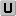Unipartite, undirected Edge typeUnweighted, multiple edges Temporal dataEdges are annotated with timestamps LoopsDoes not contain loops Join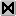Is the join of an underlying network

## Statistics

 Size n = 1,824,701 Volume m = 29,487,744 Unique edge count m̿ = 16,689,230 Loop count l = 0 Wedge count s = 490,841,289 Claw count z = 517,700,642,316 Cross count x = 260,670,210,463,189 Triangle count t = 27,718,326 Square count q = 1,911,391,423 4-Tour count T4 = 17,271,185,770 Maximum degree dmax = 7,276 Average degree d = 32.320 6 Fill p = 1.002 50 × 10−5 Average edge multiplicity m̃ = 1.766 87 Size of LCC N = 1,653,767 Diameter δ = 23 50-Percentile effective diameter δ0.5 = 5.205 37 90-Percentile effective diameter δ0.9 = 6.733 58 Median distance δM = 6 Mean distance δm = 5.744 05 Gini coefficient G = 0.713 646 Balanced inequality ratio P = 0.217 175 Relative edge distribution entropy Her = 0.942 083 Power law exponent γ = 1.653 38 Tail power law exponent γt = 2.951 00 Degree assortativity ρ = +0.114 186 Degree assortativity p-value pρ = 0.000 00 Clustering coefficient c = 0.169 413 Spectral norm α = 1,315.80 Non-bipartivity bA = 0.347 088 Normalized non-bipartivity bN = 0.024 538 3 Algebraic non-bipartivity χ = 0.044 354 8 Spectral bipartite frustration bK = 0.001 123 70

## Plots

### Degree distribution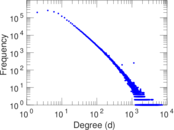### Cumulative degree distribution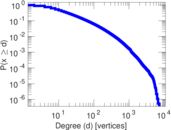### Lorenz curve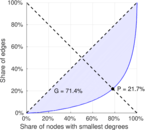### Spectral distribution of the adjacency matrix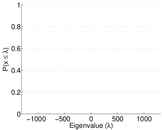### Spectral distribution of the normalized adjacency matrix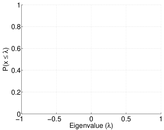### Spectral distribution of the Laplacian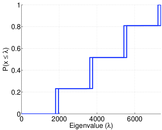### Spectral graph drawing based on the adjacency matrix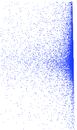### Spectral graph drawing based on the normalized adjacency matrix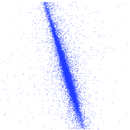### Hop distribution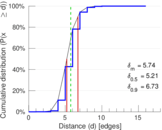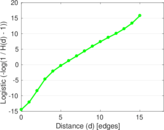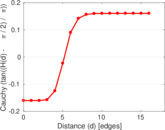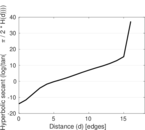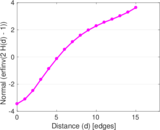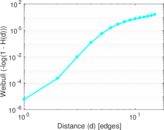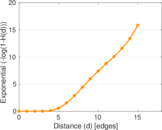### Edge weight/multiplicity distribution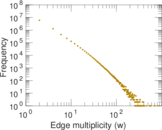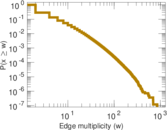### Clustering coefficient distribution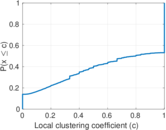### Temporal distribution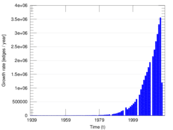### Diameter/density evolution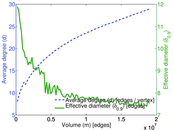### SynGraphy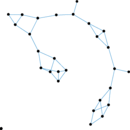### Inter-event distribution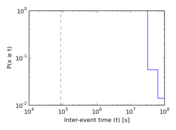### Matrix decompositions plots